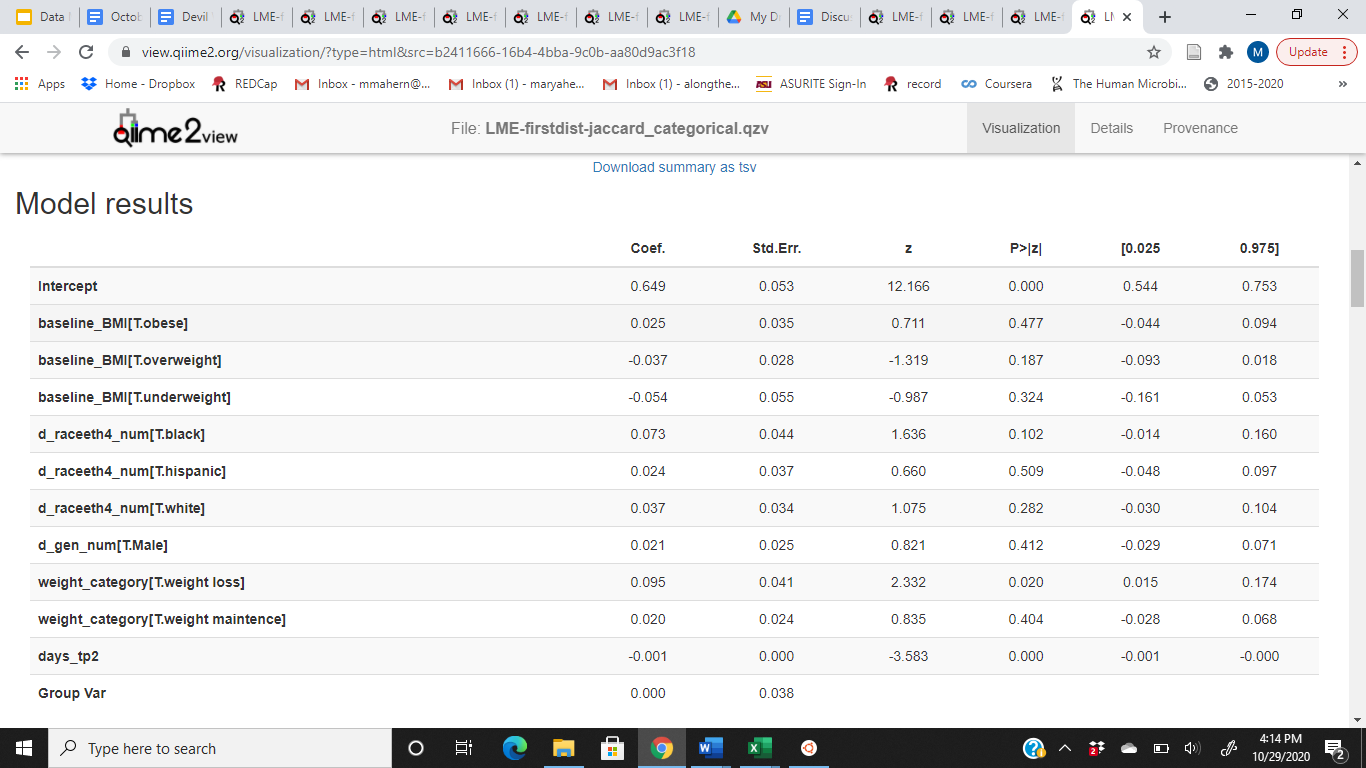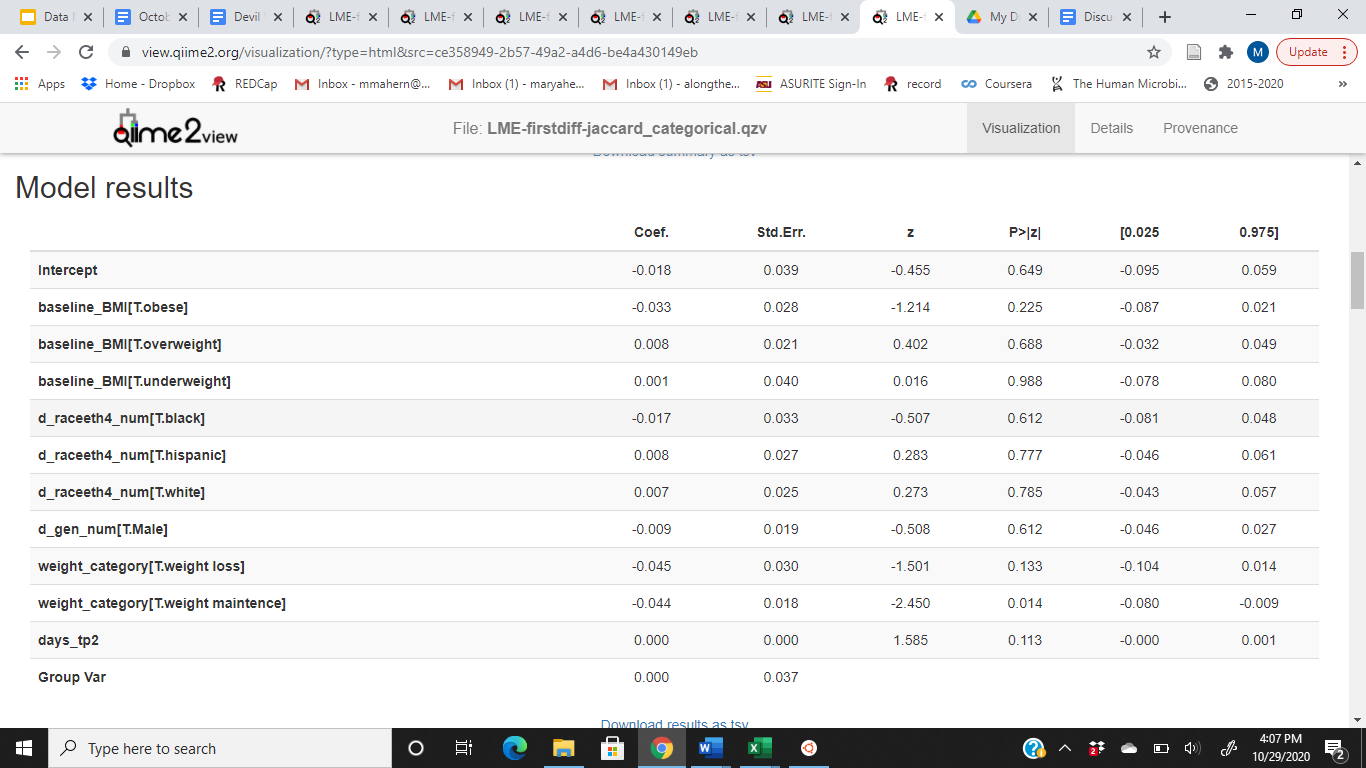# Help with First Distances and First Differences

Hi Everyone!

So I am still pretty new to qiime and microbiome analysis in general. I am at the end of my analysis but I have a question on the results and a few things I couldn't find in the forums or documents. I am interested in studying the changes in gut microbiome over time in relation to weight gain. I have a sample of about 130 college students from the beginning and the end of each semester of their freshman year. To explain some of the variables, participants were put into groups (weight loss, weight gain or weight maintenance) based on how their weight changed over the course of the year.

Among other tests, I ran first differences and first distances, then took these outputs and ran a LME model. Everything ran smoothly but now I am interpreting them and am having a hard time distinguishing the difference between first distance and first difference, especially when it comes to the beta diversity metrics. If first distances is similar to first differences just with a distance matrix as the input, then how would I interpret these results?

As an example I have attached my commands for first differences jaccard and first distances jaccard with the corresponding LME commands and results. I couldn't find any documentation that went over the units for the coefficient for first differences and distances, which is also contributing to my confusion as to how to interpret all of this. For example, are the First Distances Jaccard LME results telling me that the rate of change in the weight loss group (when compared to the reference group of weight gain) is increasing by that coefficient or that the distance between the weight loss group and weight gain group is increasing by that coefficient?

First Distances Jaccard
qiime longitudinal first-distances
--i-distance-matrix jaccard_distance_matrix.qza
--p-state-column FecalTP
--p-individual-id-column sparkid
--p-replicate-handling random
--o-first-distances jaccard-first-distancesFecalTP.qza

LME- First Distances Jaccard
qiime longitudinal linear-mixed-effects
--p-state-column days_tp2
--p-metric Distance
--p-formula Distance~days_tp2+baseline_BMI+d_raceeth4_num+d_gen_num+weight_category
--p-individual-id-column sparkid
--p-group-columns d_raceeth4_num,d_gen_num,baseline_BMI,weight_category
--o-visualization LME-firstdist-jaccard_ categorical.qzvFirst Differences Jaccard
qiime longitudinal first-differences
--p-state-column FecalTP
--p-metric "Axis 1"
--p-individual-id-column sparkid
--p-replicate-handling random
--o-first-differences jaccard-first-differencesFecalTP.qza

LME- First Differences Jaccard
qiime longitudinal linear-mixed-effects
--p-state-column days_tp2
--p-metric Difference
--p-individual-id-column sparkid
--p-group-columns baseline_BMI,d_raceeth4_num,d_gen_num,weight_category
--p-formula Difference~days_tp2+baseline_BMI+d_raceeth4_num+d_gen_num+weight_category
--o-visualization LME-firstdiff-jaccard_categorical.qzvThank you so much!!
Mary

2 Likes

Hello Mary,

Thank you for explaining your study. This sounds fascinating.I think these two examples from the longitudinal tutorial show how these methods contrast.

`first-difference` is used on alpha diversity measures (like Shannon’s index, in this example), while `first-distance` is used on beta diversity measure like (Jaccard distances in this example).

That’s also sort of mentioned in this sentence of the tutorial

A similar method [to `first-difference`] is `first-distances`, which instead identifies the beta diversity distances between successive samples from the same subject, [instead of alpha diversity differences].

Can I qiime dev to comment on using `first-differences` with Jaccard distances?``````# First Differences Jaccard
qiime longitudinal first-differences
–p-state-column FecalTP
–p-metric “Axis 1”
–p-individual-id-column sparkid
–p-replicate-handling random
–o-first-differences jaccard-first-differencesFecalTP.qza
``````

Does that help?
Colin

1 Like

Hello Mary!

If you have not already, my first advice is to use the “volatility” action to plot both the first distances and differences (you should be able to input both and view in the same visualization!). This will make the differences in behavior clearer.

In your example, you are using First Differences to look at FD in Jaccard PCoA coordinates (Axis 1), so the output is effectively telling you “how do individuals move around on Axis 1 over the course of the study?” (as in what is their rate of change at each timepoint interval, not their absolute position)

First distances is looking instead at the Jaccard distance (fraction of features not shared by each pair of samples) from the same individual at each time interval.

So these two results are tangentially related but will not necessarily yield the same results, as you are looking at position on a PC axis (i.e., after dimensionality reduction, so these coordinates are impacted by the relationship of those samples to every other sample as well) vs. what fraction of features disappear/emerge in the same individual across each interval.

This is because the units are whatever the input is — so we cannot document all possibilities! In your case:
differences: change in PC1 position
distances: Jaccard distance (fraction of unique features gained/lost at each interval in that individual)

Somewhere between those two. That the change in Jaccard distance between an individual and themselves at baseline is increased by that coefficient in the weight loss group vs. the reference group. So individuals losing weight have more species “turnover” than do those gaining weight.

A couple more tips:

you can add interaction terms, e.g., if you want to look at time:weight_category interaction

If you are using a formula, the group columns parameter is ignored so this line is unnecessary.

I hope that helps!

4 Likes

Thank you so much, that was very helpful and answered all of my questions!

2 Likes

This topic was automatically closed 31 days after the last reply. New replies are no longer allowed.